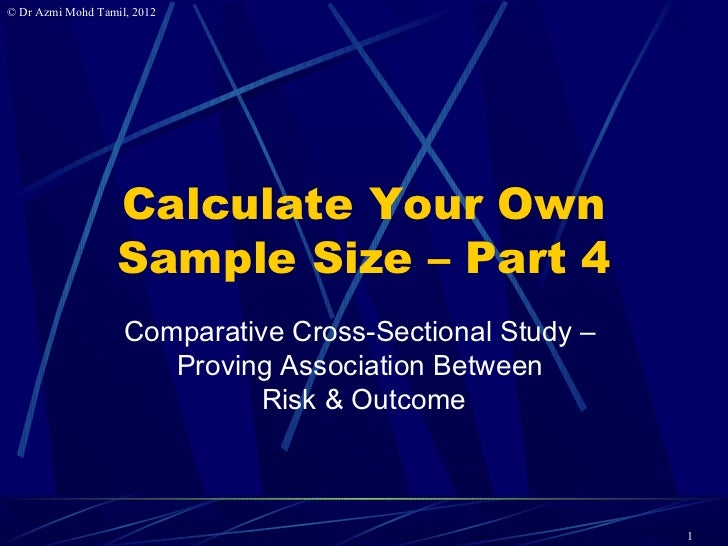Successfully reported this slideshow.Upcoming SlideShare
×

# 4. Calculate samplesize for cross-sectional studies

Part 4 of 9; Calculate samplesize for cross-sectional studies, dichotomous outcome.

• Full Name
Comment goes here.

Are you sure you want to Yes No### 4. Calculate samplesize for cross-sectional studies

1. 1. © Dr Azmi Mohd Tamil, 2012 Calculate Your Own Sample Size – Part 4 Comparative Cross-Sectional Study – Proving Association Between Risk & Outcome 1
2. 2. © Dr Azmi Mohd Tamil, 2012 Comparative Cross- Sectional What is the main objective of the study?  Association between the major risk factor being studied and the outcome? 2
3. 3. © Dr Azmi Mohd Tamil, 2012 Cross-Sectional: Risk & Outcome If you want to show an association between a risk factor and outcome in a cross-sectional study, then the sample size calculation is different. For example I want to prove that Indians (ethnicity = risk) are at higher risk of having diabetes mellitus (outcome) compared to other races in Malaysia, using a cross- sectional study. 3
4. 4. © Dr Azmi Mohd Tamil, 2012 Example – Indians in M’sia are of higher risk for DM From literature review, identify the rate of disease and proportion of those with the risk factor. Proportion of sample from unexposed (Others) population = 85% Proportion of sample from exposed (Indians) population = 15% P1=true proportion of DM in unexposed (Others) population = 8% P2=true proportion of DM in exposed (Indians) population =14% 4
5. 5. © Dr Azmi Mohd Tamil, 2012 From Literature Review: Indians & Diabetes M. Disease + (14%) Indians (15%) Disease - (86%) Sample ratio Disease + (8%) Others (85%) Disease - (92%) 5
6. 6. © Dr Azmi Mohd Tamil, 2012 Calculate Manually Calculate using these formulas (Fleiss JL. 1981. pp. 44-45) m=n1=size of sample from population 1 n2=size of sample from population 2 P1=proportion of disease in population 1 P2=proportion of disease in population 2 α= "Significance” = 0.05 β=chance of not detecting a difference = 0.2 1-β = Power = 0.8 r = n2/n1 = ratio of cases to controls P = (P1+rP2)/(r+1) Q = 1-P. n1 = m n2 = rm From table A.2 in Fleiss; If 1- α is 0.95 then cα/2 is 1.960 If 1- β is 0.80 then c1-beta is -0.842 6
7. 7. © Dr Azmi Mohd Tamil, 2012 Calculate Manually 7
8. 8. Or use StatCalc© Dr Azmi Mohd Tamil, 2012 n1 = 1422 n2 = 251 Total sample size required is 1673 8
9. 9. © Dr Azmi Mohd Tamil, 2012 StatCalc = Manual Sample size calculated manually is similar to the sample size generated by StatCalc. Since both methods use Fleiss JL (1981) unequal sample size formula as shown in the next slide. 9
10. 10. © Dr Azmi Mohd Tamil, 2012 10
11. 11. © Dr Azmi Mohd Tamil, 2012 Or we can use PS2 Based on this, the sample size for exposed is 231. So unexposed is 85/15*231= 1309 Total 1540 11
12. 12. © Dr Azmi Mohd Tamil, 2012 PS2 ≠ StatCalc PS2 uses Schlesselman’s method for independent case and control groups for studies that will be analysed using an uncorrected chi-square test PS2 uses Casagrande et al’s method for independent studies that will be analysed using Yates Correction or Fishers exact test. PS2 only uses the generalisation of Casagrandes method proposed by Fleiss for unequal case & control sample size. Even then the answers differ; 1673 vs 1540 12
13. 13. © Dr Azmi Mohd Tamil, 2012 What If There Is No Prior Information? Instead of saying "Sample sizes are not provided because there is no prior information on which to base them“, do this instead;  Find previously published information  Conduct small pre-study  If a very preliminary pilot study, sample size calculations not usually necessary 13
14. 14. © Dr Azmi Mohd Tamil, 2012 Conclusion You can calculate your own sample size. Tools are available and most of them are free. Decide what is your study design and choose the appropriate method to calculate the sample size. If despite following ALL these notes fastidiously, your proposal is still rejected by the committee due to sample size, kindly SEE THEM, not us. 14
15. 15. © Dr Azmi Mohd Tamil, 2012 References (incl. for StatCalc) Fleiss JL. Statistical methods for rates and proportions. New York: John Wiley and Sons, 1981. Gehan EA. Clinical Trials in Cancer Research. Environmental Health Perspectives Vol. 32, pp. 3148, 1979. Jones SR, Carley S & Harrison M. An introduction to power and sample size estimation. Emergency Medical Journal 2003;20;453-458. 2003 Kish L. Survey sampling. John Wiley & Sons, N.Y., 1965. Krejcie, R.V. & Morgan, D.W. (1970). Determining sample size for research activities. Educational & Psychological Measurement, 30, 607-610. Snedecor GW, Cochran WG. 1989. Statistical Methods. 8th Ed. Ames: Iowa State Press. 15
16. 16. © Dr Azmi Mohd Tamil, 2012 References (PS2) Dupont WD, Plummer WD, Jr: Power and Sample Size Calculations: A Review and Computer Program. Controlled Clinical Trials 11:116-128, 1990 Dupont WD, Plummer WD, Jr: Power and Sample Size Calculations for Studies Involving Linear Regression. Controlled Clinical Trials 19:589-601, 1998 Schoenfeld DA, Richter JR: Nomograms for calculating the number of patients needed for a clinical trial with survival as an endpoint. Biometrics 38:163-170, 1982 Pearson ES, Hartley HO: Biometrika Tables for Statisticians Vol. I 3rd Ed. Cambridge: Cambridge University Press, 1970 Schlesselman JJ: Case-Control Studies: Design, Conduct, Analysis. New York: Oxford University Press, 1982 Casagrande JT, Pike MC, Smith PG: An improved approximate formula for calculating sample sizes for comparing two binomial distributions. Biometrics 34:483-486, 1978 Dupont WD: Power calculations for matched case-control studies. Biometrics 44:1157-1168, 1988 Fleiss JL. Statistical methods for rates and proportions. New York: John Wiley and Sons, 1981. 16
17. 17. © Dr Azmi Mohd Tamil, 2012 THANK YOU